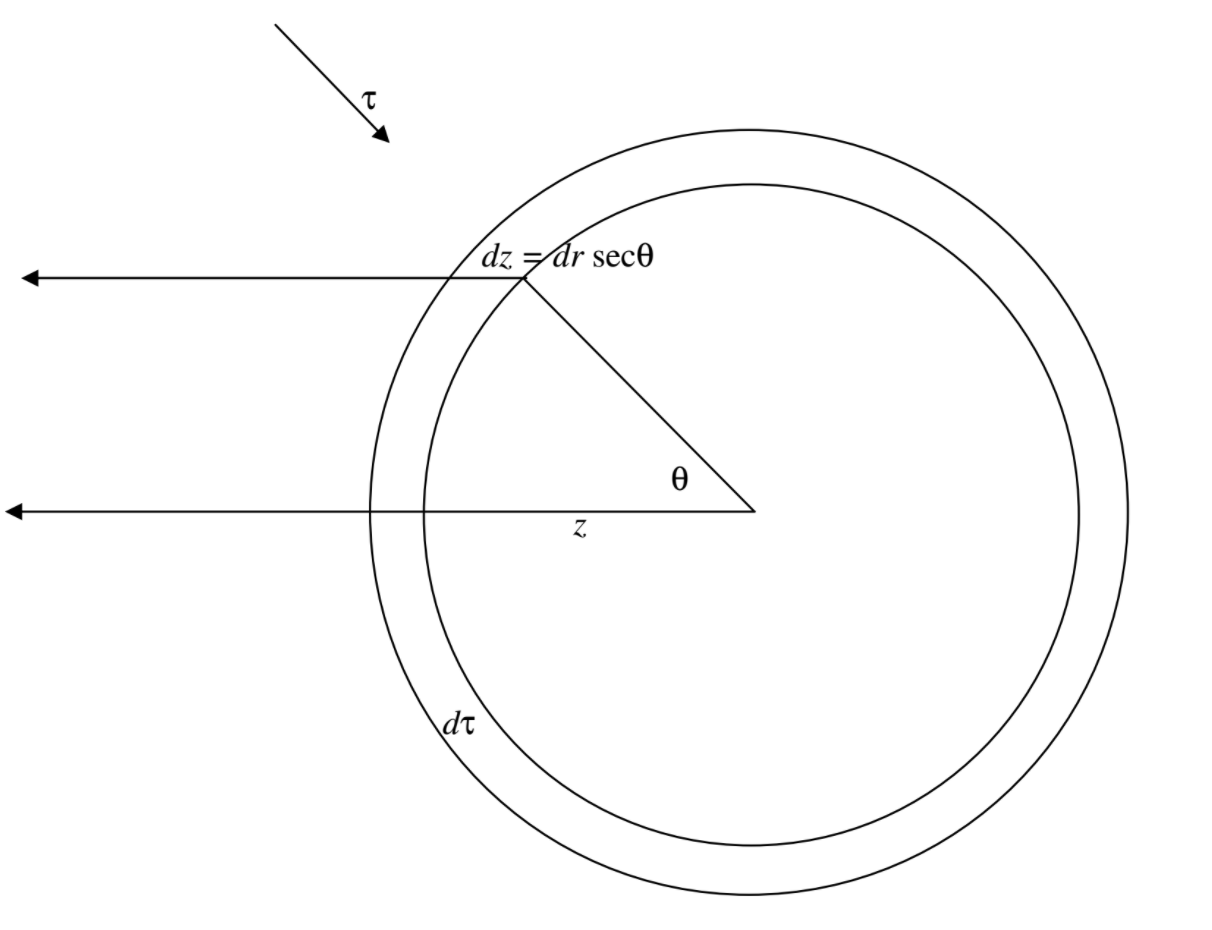# 5.9: More on the Equation of Transfer

$$\newcommand{\vecs}{\overset { \rightharpoonup} {\mathbf{#1}} }$$ $$\newcommand{\vecd}{\overset{-\!-\!\rightharpoonup}{\vphantom{a}\smash {#1}}}$$$$\newcommand{\id}{\mathrm{id}}$$ $$\newcommand{\Span}{\mathrm{span}}$$ $$\newcommand{\kernel}{\mathrm{null}\,}$$ $$\newcommand{\range}{\mathrm{range}\,}$$ $$\newcommand{\RealPart}{\mathrm{Re}}$$ $$\newcommand{\ImaginaryPart}{\mathrm{Im}}$$ $$\newcommand{\Argument}{\mathrm{Arg}}$$ $$\newcommand{\norm}{\| #1 \|}$$ $$\newcommand{\inner}{\langle #1, #2 \rangle}$$ $$\newcommand{\Span}{\mathrm{span}}$$ $$\newcommand{\id}{\mathrm{id}}$$ $$\newcommand{\Span}{\mathrm{span}}$$ $$\newcommand{\kernel}{\mathrm{null}\,}$$ $$\newcommand{\range}{\mathrm{range}\,}$$ $$\newcommand{\RealPart}{\mathrm{Re}}$$ $$\newcommand{\ImaginaryPart}{\mathrm{Im}}$$ $$\newcommand{\Argument}{\mathrm{Arg}}$$ $$\newcommand{\norm}{\| #1 \|}$$ $$\newcommand{\inner}{\langle #1, #2 \rangle}$$ $$\newcommand{\Span}{\mathrm{span}}$$$$\newcommand{\AA}{\unicode[.8,0]{x212B}}$$

Refer to equation 5.5.1. We see from what had been subsequently discussed that $$[\alpha(\nu) + \sigma(\nu)]dx = d\tau(\nu)$$ and that $$j_\nu dx = d\tau(\nu)$$. Therefore

$\frac{dI_v}{d\tau(\nu)} = S_\nu - I_\nu , \label{5.9.1}$

and this is another form of the equation of transfer.

Now consider a spherical star with a shallow atmosphere ("plane parallel atmosphere"). In figure $$\text{V.6}$$, radial distance $$r$$ is measured radially outwards from the centre of the star. Optical depth is measured from outside towards the centre of the star. The thickness of the layer is $$dr$$. The coordinate $$z$$ is measured from the centre of the star towards the observer, and the path length through the atmosphere in that direction at angle $$\theta$$ is $$dz = dr \sec \theta$$. The equation of transfer can be written

$dI_\nu (\theta) = - [\kappa (\nu) I_\nu (\theta) - j_\nu] dz. \label{5.9.2}$

Now $$\kappa (\nu) dz = -\sec \theta d\tau(\nu)$$ and $$j_\nu = \kappa (\nu) S_\nu$$. Therefore

$\cos \theta \frac{dI_{\nu}(\theta)}{d\tau (\nu)} = I_{\nu} (\theta) - S_\nu \label{5.9.3}$

This is yet another form of the equation of transfer. The quantity $$\cos\theta$$ is often written $$\mu$$, so that equation $$\ref{5.9.3}$$ is often written

$\mu\frac{dI_v(\theta)}{d\tau(\nu)} = I_\nu(\theta) - S_\nu \label{5.9.4}$$$\text{FIGURE V.6}$$

Let us do $$\frac{1}{4\pi} \oint d\omega$$ to each term in equation $$\ref{5.9.4}$$. By $$\oint$$ I mean integrate over $$4\pi$$ steradians. In spherical coordinates $$d \omega = \sin \theta d \theta d \phi$$. We obtain

$\frac{1}{4\pi} \oint \frac{dI_\nu (\theta)}{d \tau(\nu)} \cos \theta d \omega = \frac{1}{4 \pi} \oint I_\nu d \omega - \frac{1}{4 \pi} \oint S_\nu d \omega \label{5.9.5}$

The left hand side is $$dH_\nu/d\tau(\nu)$$ and the first term on the right hand side is $$J_\nu$$. (See the definitions - equations 4.5.2 and 4.7.1.) In the case of isotropic scattering, the source function is isotropic so that, in this case

$\frac{dH_\nu}{d\tau(\nu)} = J_\nu - S_\nu, \label{5.9.6}$

and this is another form of the equation of transfer.

On the other hand, if we do $$\frac{1}{4\pi} \oint \cos \theta d \omega$$ to each term in equation 5.15, we obtain

$\frac{1}{4\pi} \oint \frac{dI_\nu(\theta)}{d\tau(\nu)} \cos^2 \theta d \omega = \frac{1}{4\pi} \oint I_\nu \cos \theta d \omega - \frac{1}{4\pi} \oint S_\nu \cos \theta d \omega \label{5.9.7}$

In the case of isotropic scattering the last integral is zero, so that

$\frac{dK_\nu}{d\tau(\nu)} = H_\nu , \label{5.9.8}$

and this is yet another form of the equation of transfer.

Now $$H_\nu$$ is independent of optical depth (why? - in a plane parallel atmosphere, this just expresses the fact that the flux (watts per square metre) is conserved), so we can integrate equation $$\ref{5.9.8}$$ to obtain

$K_\nu = H_\nu \tau(\nu) + \text{constant} \label{5.9.9}$

Note also that $$H_\nu = F_\nu /(4\pi)$$, and, if the radiation is isotropic, $$K_\nu = J_\nu/ 3$$ so that,

$J_\nu = \frac{3 F_\nu \tau(\nu)}{4\pi} + J_\nu(0) \label{5.9.10}$

where $$J_\nu(0)$$ is the mean specific intensity (radiance) at the surface, which is half the specific intensity at the surface (since the radiance of the sky above the surface is zero). Thus

$J_\nu (0) = \frac{1}{2} I_\nu (0) = F_\nu / (2\pi) \label{5.9.11}$

Therefore

$J_\nu = \frac{F_\nu}{2\pi} \left( 1 + \frac{3}{2} \tau ( \nu ) \right) \label{5.9.12}$

This shows, to this degree of approximation (which includes the approximation that the radiation in the atmosphere is isotropic - which can be the case exactly only at the centre of the star) how the mean specific intensity increases with optical depth.

Let $$T$$ be the temperature at optical depth $$\tau$$.

Let $$T_0$$ be the surface temperature.

Let $$T_{\text{eff}}$$ be the effective temperature, defined by $$F(0) = \sigma T^4_{\text{eff}}$$,

We also have $$\pi J = \sigma T^4$$ and $$\pi J (0) = \sigma T_0^4 = \frac{1}{2} F$$.

From these we find the following relations between these temperatures:

$T^4 = \left( 1 + \frac{3}{2} \tau \right) T^4_0 = \frac{1}{2} \left( 1 + \frac{3}{2} \tau \right) T^4_{\text{eff}} \label{5.9.13}$

$T_0^4 = \frac{2}{2+3\pi}T^4 = \frac{1}{2} T^4_{\text{eff}} \label{5.9.14}$

$T^4_{\text{eff}} = \frac{4}{2+3\tau}T^4 = 2 T^4_0 \label{5.9.15}$

Note also that $$T = T_{\text{eff}}$$ at $$\tau = 2/3$$, and $$T = T_0$$ at $$\tau = 0$$.

This page titled 5.9: More on the Equation of Transfer is shared under a CC BY-NC 4.0 license and was authored, remixed, and/or curated by Jeremy Tatum via source content that was edited to the style and standards of the LibreTexts platform; a detailed edit history is available upon request.